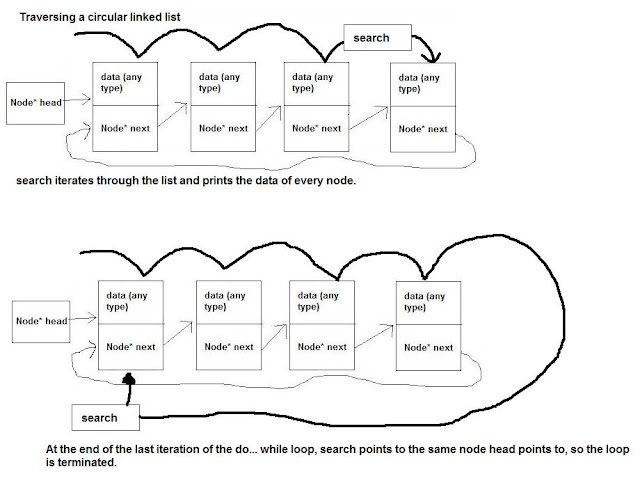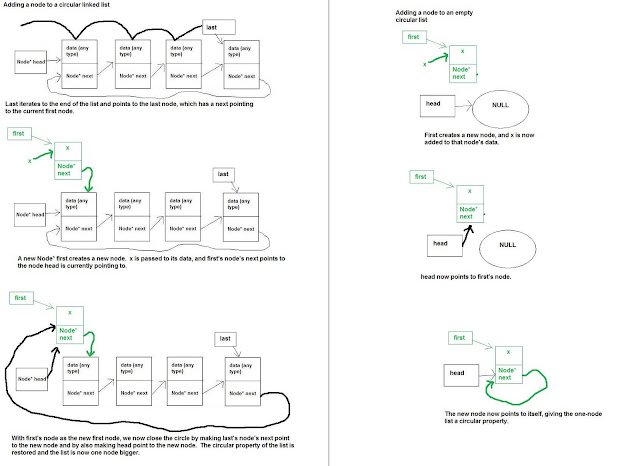## Wednesday, September 8, 2010

### Lesson 2. Circular linked lists

If you recall, the Node* of the last node of a singly-linked list points to null. However, it doesn’t necessarily have to point to null. You can make a singly-linked list circular instead of linear by making the last node of the list point to the first node of the list. It looks like this.So how do you make the last node’s Node* point to the first node? Well, what else is pointing to the first node?

So what you do is every time you alter the linked list by doing something like inserting or removing nodes at the head of the list, you set the last node’s Node* equal to head so they both point to the first node.

Here’s what the class for a circular linked list looks like:

class CircleList

{
public:
CircleList();
bool isEmpty();
void circleTraverse();
void circleInsert(int x);
int circleRemove();
~CircleList();
};

Note: circular linked lists use the same node struct as linear singly-linked lists.

The constructor looks like this:

CircleList::CircleList()
{
}

which is exactly the same as the constructor for a linear singly-linked list.

This is the traverse function.

void CircleList::circleTraverse()

{
if(!isEmpty())
{
do{
cout<< search -> data << endl;
search = search -> next;
}
}

In this function, no comments were added because the only real difference between traversing a circular linked list and a linear linked list is the use of a do… while loop. In the function, the search node starts out at the head of the list and enters the do… while loop if the list is not empty. With each iteration of the do… while loop, the search Node* prints the data of the node that search points to and then points to the next node in the list. At the last node’s iteration, search points to that node’s next, which is also the first node, which is also the node head points to, so search is now equal to head and search != head evaluates to false, ending the loop.Just for comparison, here’s the linear linked list’s traverse function.

{
while(search != NULL)
{
cout<< search -> data << endl;
search = search -> next;
}
}

Now, here’s the new insert function.

void CircleList::circleInsert(int x)

{
if(isEmpty())
{
}
else
{
do{
last = last -> next;//3
Node* first = new Node;
first -> data = x;
}
}

1. If the list is empty, the head now points to the new node being added, and the Node* of the new node points to the node head is pointing to, which is the new node, so the new node is linked to itself, giving this one-item circleList its circular property.

2. Otherwise, we will need to traverse the list to get to the last node. To do this we create a pointer to the last node.

3. Like in the traverse code, we use a do… while loop to get to the end since last starts out pointing to head. Once we are at the last node, the loop ends.

4. A new node called first is created to be the new first node, holding the data passed to the function and pointing to the node head is pointing to, which is the old first node. After this, head points to the new first node.

5. Last’s Node* next now points to the new first node, restoring the circular linked list property.

As you can see, to keep the circular property of the linked list there was a trade-off in writing the insert function for a circular linked list. For example, there are extra lines of code added for keeping the circular property. The most notable change, though, is the increased runtime. To get to the last node in order to retain the circular property of the list, we had to iterate through the whole list, giving the function a worst-case runtime of O(n), which is still good, but slow compared to the linear linked list’s O(1) runtime for insertion.Now let’s look at the remove function.

//Invariant: circleRemove is not to be used on an empty list.

int CircleList::circleRemove()
{
int x = temp -> data;
{
delete temp;
}
else
{
do{
last = last -> next;
delete temp;//6
}

return x;
}

1. Like in remove, you have to follow the remove function’s invariant that it will never be used on an empty list, so the code assumes the list is not empty.

2. If temp -> next is pointing to the same node head points to, temp -> next is pointing to temp, so there is only one node in the list. Because of this, what the function will do is remove the one node in the list, make the list empty, and then return the one node’s data.

3. If there is more than one node in the list, then the list will not be empty after the node is deleted, so the circular property must be retained. Because of this, once again we need to traverse the list to the last node using a do… while loop, bringing the runtime of the function to O(n).

4. Head now points to the second node in the list.

5. Last now points to the node head now points to, which is the second node in the list, restoring the circular property and making the second node into the new first node.

6. Temp is deleted and its data are returned.

Also, once again it is notable that maintaining a circular property requires you to make the remove function longer to write, and longer to run (O(1) to O(n)) due to the traversal step.Finally, here is the destructor for a circular linked list:

CircleList::~CircleList()
{
{
circleRemove();
}
}

Really, there’s nothing that notable to point out when you consider that the destructor is pretty much exactly the same for circular linked lists as it is for linear linked lists.

insertIth and removeIth are not going to be shown in this tutorial because the only thing that really changes is that now you don’t need to make a special case for inserting or removing at the last position since there is no node pointing to NULL in the list. However, I will tell you that with insertIth and removeIth, since the circular list property makes it so that the list wraps around to position 0 after passing the last element of the list, there is no longer a maximum position you need to worry about for inserting and removing items.

#### 1 comment :

1.Thanks for taking the time to discuss this, I feel strongly about it and love learning more on this topic. If possible, as you gain expertise, would you mind updating your blog with extra information? It is extremely helpful for me. linkedin ads tips